## Deep Learning with Keras and TensorFlow

Certification Training
6548 Learners
7 Chapters +

# How to Train an Artificial Neural Network

Let us begin with the objectives of this lesson.Welcome to the third lesson ‘How to train an Artificial Neural Network’ of the Deep Learning Tutorial, which is a part of the Deep Learning (with TensorFlow) Certification Course offered by Simplilearn. This lesson gives you an overview of how an artificial neural network is trained.

## Objectives

After completing this lesson on ‘How to train an Artificial Neural Network’ you’ll be able to:

• Understand how ANN is trained using Perceptron learning rule.

• Explain the implementation of Adaline rule in training ANN.

• Describe the process of minimizing cost functions using Gradient Descent rule.

• Analyze how learning rate is tuned to converge an ANN.

• Explore the layers of an Artificial Neural Network(ANN).

## Artificial Neural Networks (ANN) - Definition

“Artificial Neural Network is a computing system made up of a number of simple, highly interconnected processing elements which process information by their dynamic state response to external inputs.” - Robert Hecht-Nielsen.

## Layers of ANN

The diagram shows a three-layered neural network: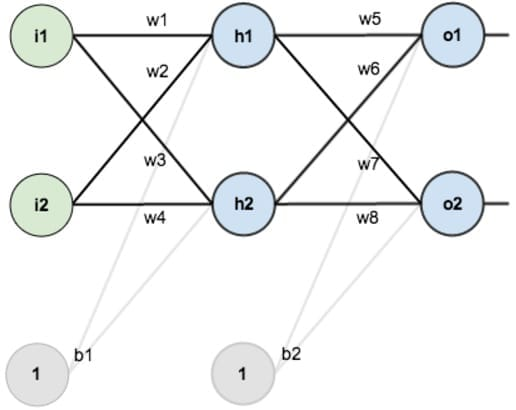The layers are:

• Input layer

• Output layer

• One hidden layer.

Input for the hidden layer

The input for hidden layer neuron is weighted outputs of input neurons plus a bias term.

Total net input for h1

neth1 = w1*i1 + w2*i2 + b1*1

Output for the hidden layer

The output of the hidden layer passes through a sigmoid transformation using the sigmoid activation function. The output of a sigmoid is a value between 0 and 1.

Finally, the outputs of the hidden layer are again weighted to produce the output layer values o1 and o2.

Output of h1 :-

(Apply sigmoid activation function)

outh1 = 1/(1+e-neth1)

## How to Train Artificial Neural Networks (ANN)

Single layer neural network (or perceptrons) can be trained using either the Perceptron training rule or the Adaline rule.

Perceptron Training Rule (Rosenblatt’s Rule):

• Works well when training samples are linearly separable

• Updates weights based on the error in the threshold perceptron output (e.g.: +1 and -1)

• Works well even when the training samples are not linearly separable

• Makes necessary changes to the template

• Updates weights based on the error in the non-threshold linear combination of outputs

• Converges towards the best-fit approximation of the target output

• Provides a basis for backpropagation algorithm, which can learn networks with many interconnected units

## Perceptron Learning Rule (Rosenblatt’s Rule)

A perceptron is a computational unit that calculates the output based on weighted input parameters.

### Steps To Follow

The basic idea is to mimic how a single neuron in the brain works: it either fires or it doesn't. Rosenblatt's initial perceptron rule is fairly simple and can be summarized by the following steps:

• Initialize the weights to 0 or small random numbers.

• For each training sample x(i): Compute the output value y ̂.

• Update the weights.

Here the output value is the predicted class label produced by the unit step function.

Weight adjustment at each step is written as: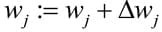The value of weight change is calculated as: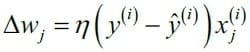Here “η” is the learning rate (typically a constant between 0.0 and 1.0)

“y(i)” is the true class label

“y ̂(i)” is the predicted class label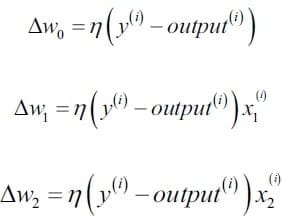It is important to note that all weights in the weight vector are being updated simultaneously. Hence for a two-dimensional dataset, the weight update would be:

### Prediction Of The Class Label

Case 1: Perceptron predicts the class label correctly.

• The weights remain unchanged.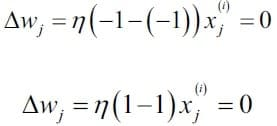Case 2: Perceptron predicts the class label wrongly.

The weights are being pushed towards the direction of the positive or negative target class.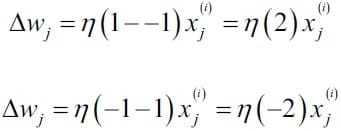### Convergence In Neural Network

Convergence is performed so that cost function gets minimized and preferably reaches the global minima. It is also done to find the best possible weights to minimize the classification problem.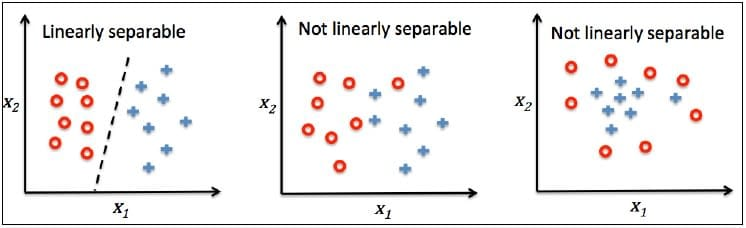Convergence of the learning algorithms is guaranteed only if:

• The two classes are linearly separable

• The learning rate is sufficiently small

NOTE: If a linear decision boundary can't separate the two classes, you can set a maximum number of passes over the training dataset (epochs) and/or a threshold for the number of tolerated misclassifications. The perceptron would never stop updating the weights otherwise.

Overall, the perceptron rule can be summed up to the following points:

• The perceptron receives the inputs of sample x and combines them with the weights w to compute the net input.

• The net input is then passed on to the threshold function, which generates a binary output -1 or +1: the predicted class label of the sample.

• During the learning phase, this output is used to calculate the error of the prediction and update the weights.

Willing to take up a course in Deep Learning? Check out our Deep Learning Course Preview now!

In Adaline, the weights are updated based on a linear activation function.

The linear activation function φ(z) is the identity function of the net input, so that:

φ(wTx) = wTx

While the linear activation function is used for learning the weights, a threshold function is used to make the final prediction, which is similar to the unit step function.

The Adaline Rule and Perceptron Rule can be differentiated as follows: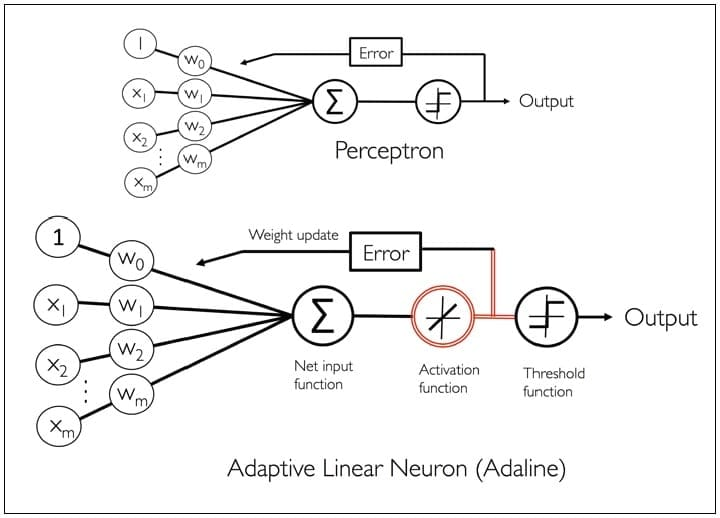Adaline Rule:

In this rule -

• Weights are updated based on a linear activation function

• Compares the true class labels with the linear activation function's continuous valued output to compute the model error and update the weights.

Perceptron Rule

In this rule:

• Weights are updated based on a unit step function.

• Compares the true class labels with the predicted class labels to compute the model error and update the weights.

### Minimizing Cost Functions

The most common neural networks belong to supervised learning category, where ground truth output labels are available for training data. One key technique in supervised learning is to optimize an objective function, which enables the learning process.

This objective function is often a cost function which is to be minimized.

### Sum Of Squared Errors(Sse)

In Adaline, Sum of Squared Errors (SSE) is the cost function J which needs to be minimized. SSE is a squared difference of calculated outcomes and true class labels.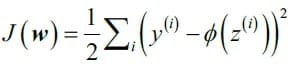Minimizing this brings the predicted output close to ground truth labels. Also, squaring makes it differentiable.

The main advantage of this continuous linear activation function, in contrast to the unit step function, is that the cost function becomes differentiable and convex.

Hence, a simple yet powerful optimization algorithm called Gradient Descent can be used to find the weights that minimize the cost function to classify the samples in the dataset.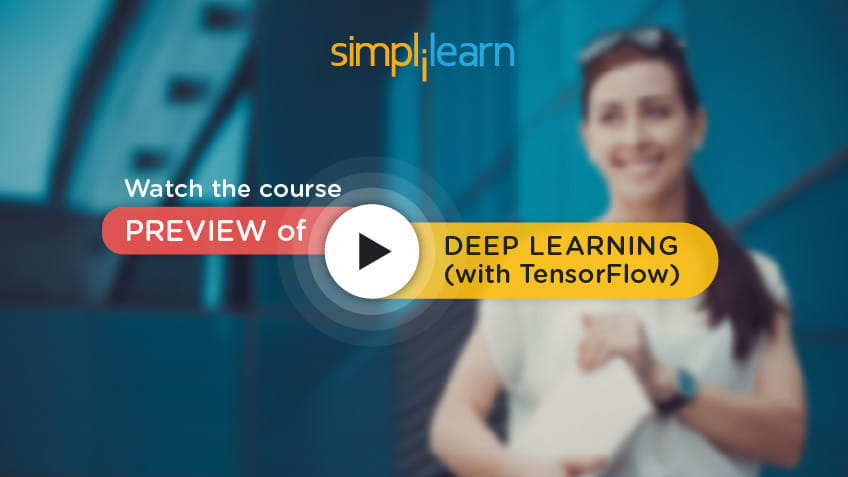Minimizing Cost Functions With Gradient Descent

The main idea behind gradient descent is to go down the hill of cost function until a local or global minimum point is reached.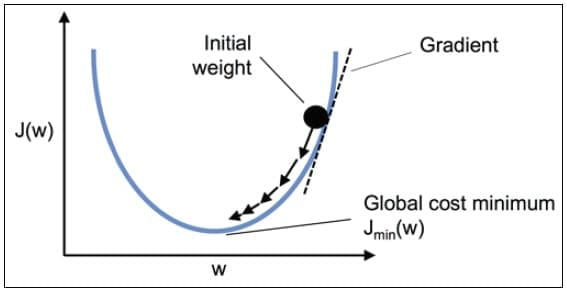In each iteration, a step is taken in the opposite direction of the gradient where the step size is determined by the value of the learning rate.

## Steps to Minimize Cost Functions With Gradient Descent

Using gradient descent, update weight by taking a step in the opposite direction of the gradient.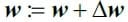The weight change “Δw” is defined as the negative gradient multiplied by the learning rate “η”.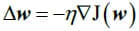To compute the gradient of the cost function, compute the partial derivative of the cost function with respect to each weight “wj ”.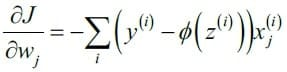So it can be written as: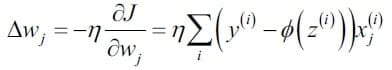Since all weights are updated simultaneously, the Adaline learning rule becomes:The partial derivative of the SSE cost function with respect to the jth weight can be obtained as shown: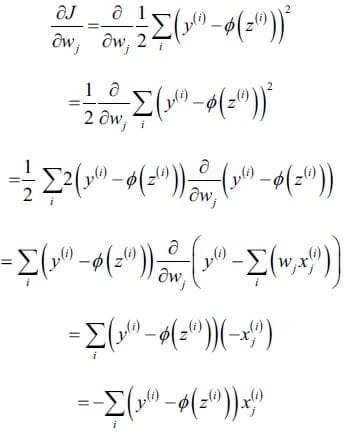## Difference Between Perceptron and Gradient Descent Rule

Both perceptron and gradient descent seem to use the rule:

But in reality the rules are different:

Perceptron Rule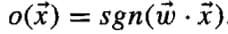• o refers to the threshold output

• The threshold output is not differentiable.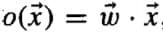• o refers to the linear unit output

• The non-threshold output is differentiable.

A logistic regression model (core Machine Learning) is closely related to Adaline with the only difference being its activation and cost function.

• Converging to local minima is very slow. (thousands of gradient descent steps needed)

• In case of multiple local minima, global minima may not be found.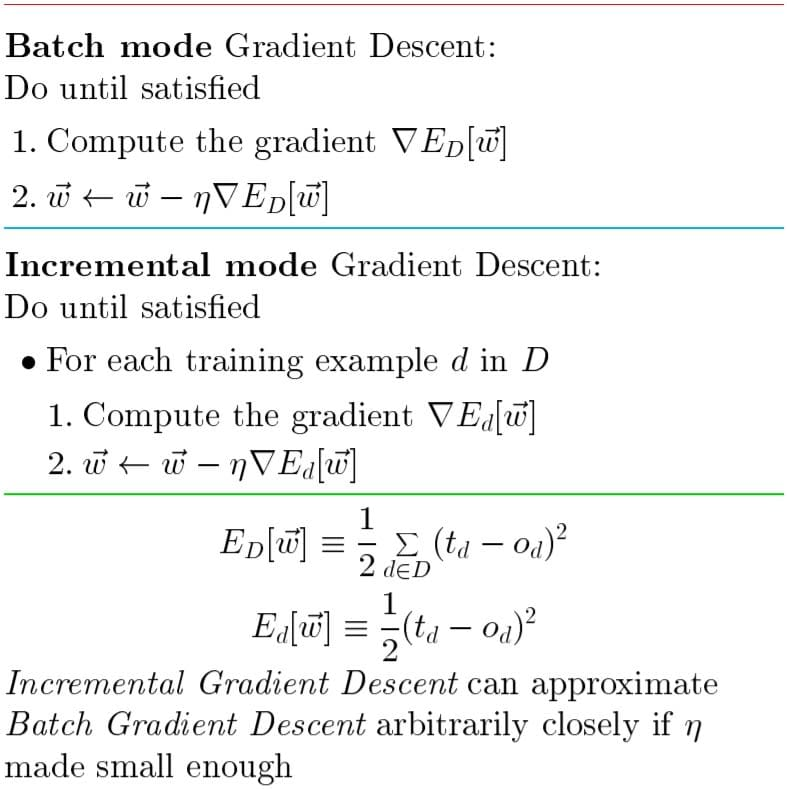These issues can be alleviated with stochastic gradient descent:

In this, weights are updated incrementally, after error calculation for each sample d, rather than computing weight updates after summing errors over all samples of D.

In the case of multiple local minima, stochastic gradient descent is a better choice to find the global minimum.

## Tune the Learning Rate

Let us learn how to tune the learning rate in the following topics.

### Hyperparameters

Hyperparameters are parameters set by the data scientist/developer while building the model based on experience or by hit and trial.

These parameters are not among those (unlike weights and biases) that get learned during training.

The hyperparameters of the perceptron and Adaline learning algorithms are:

• Learning rate “η” (eta) and

• Number of epochs (n_iter)

The learning rate indicates the speed of learning, or a factor to moderate the rate of weight adjustment over multiple training loops.

An Epoch refers to one complete training pass or one pass of the training loop.

### Optimal Convergence

In practice, it often requires some experimentation to find a good learning rate η for optimal convergence. So, let’s choose two different learning rates, η = 0.1 and η = 0.0001, to start with.

Plot the cost functions versus the number of epochs to see how well the Adaline implementation learns from the training data.

Two different types of problem are encountered.

This chart shows what could happen if a learning rate that is too large is chosen. Instead of minimizing the cost function, the error becomes larger in every epoch, because we overshoot the global minimum.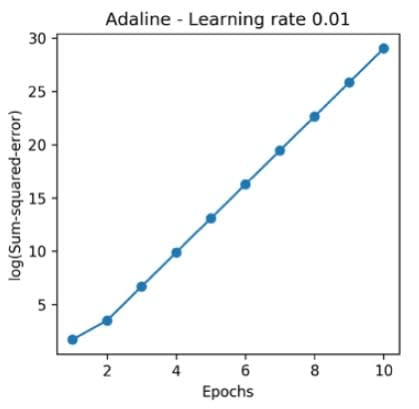η = 0.1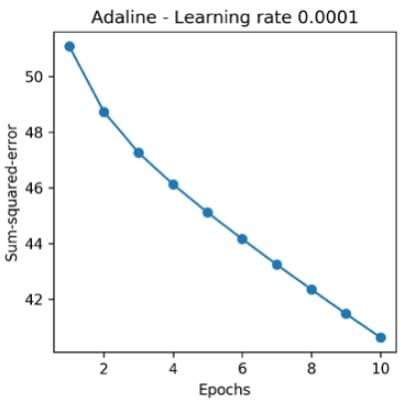η = 0.0001

In this chart, it is seen that the cost decreases, but the chosen learning rate is so small that the algorithm would require a very large number of epochs to converge to the global cost minimum.

### Convergence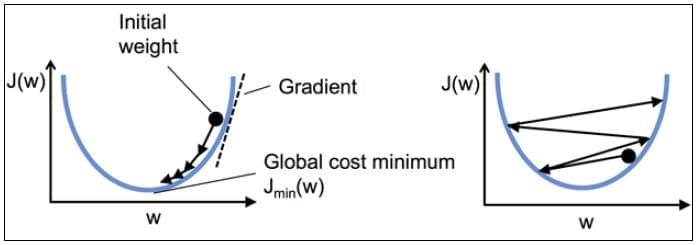The figure on the left demonstrates optimum learning rate, where the cost function converges to a global minimum.

The figure on the right shows a large learning rate, and the global minimum gets missed during weight adjustments.

## Summary

Let us summarize what we have learned in this lesson:

• Perceptron learning rule is best suited when the learning samples are linearly separable. The weights are updated based on a unit step function.

• In Adaline rule, weights are updated based on a linear activation function. They can be used even when the learning samples are not linearly separable.

• Gradient descent rule, often used as part of Adaline algorithm, can be used to find the weights that minimize the cost function.

• Selecting a large learning rate causes a large error and the global minimum gets missed during weight adjustments. Smaller learning rate ensures that the cost function converges to a global minimum.

• The artificial neural network has an input, output and a hidden layer. The output of the hidden layer is obtained by applying the sigmoid or some other activation function.

## Conclusion

This concludes the lesson “How to Train an Artificial Neural Network.” The next lesson is “Multilayer ANN.

### Find our Deep Learning with Keras and TensorFlow Online Classroom training classes in top cities:

Name Date Place
Deep Learning with Keras and TensorFlow 13 Feb -7 Mar 2021, Weekend batch Your City View Details
Deep Learning with Keras and TensorFlow 13 Mar -4 Apr 2021, Weekend batch New York City View Details
Deep Learning with Keras and TensorFlow 17 Apr -9 May 2021, Weekend batch San Francisco View Details
Related Courses
Learner Reviews
Related Articles
• Disclaimer
• PMP, PMI, PMBOK, CAPM, PgMP, PfMP, ACP, PBA, RMP, SP, and OPM3 are registered marks of the Project Management Institute, Inc.

Name*
Email*
Phone Number*